Traveltime approximations for transversely isotropic media with an inhomogeneous backgroundNext: Expansion in terms of Up: Alkhalifah: TI traveltimes in Previous: Introduction

# The TI eikonal and expansion inIn VTI media, the eikonal equation (Alkhalifah, 1998) in the acoustic approximation has the form: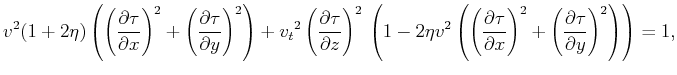(1)

whereis the traveltime (eikonal) measured from the source to a point with the coordinates, andand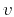are the velocity and NMO velocity (=), respectively, described with respect to the symmetry direction at that point. To formulate a well-posed initial-value problem for equation 1, it is sufficient to specifyat some closed surface and to choose one of the two solutions: the wave going from or toward the source. The level of nonlinearity in this quartic (in terms of) equation is higher than that for the isotropic or elliptically anisotropic eikonal equations. This results in much more complicated finite-difference approximations of the VTI eikonal equation.

For a tilted TI medium, the traveltime derivatives in equation 1 are taken with respect to the tilt direction, and thus, we have to rotate the derivatives in equation 1 using the following Jacobian in 3D: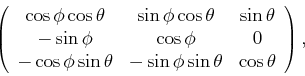(2)

to obtain an eikonal equation corresponding to the conventional computational coordinates governed by the acquisition surface. In equation 2,is the angle of the symmetry axis measured from the vertical andcorresponds to the azimuth of the vertical plane that contains the symmetry axis measured from the-axis (the axis of the source-receiver direction). Setting initially=0, for simplicity, allows us to obtain the eikonal equation for 2D TI media given by:(3)

The full 3D version of this equation is stated in Appendix D.

scanTheta
Figure 1.
A schematic plot showing the relation between a background traveltime field for=0 and that whenis larger than zero. The round dot at the top of theplane represents a source.Solving equation 3 numerically requires solving a quartic equation (instead of the quadratic in the isotropic and elliptical anisotropic case) at each computational step. Alternatively, it can be solved using perturbation theory (Bender and Orszag, 1978) by approximating equation 3 with a series of simpler linear equations. Consideringconstant and small, we can represent the traveltime solution as a series expansion in. This will result in a solution that is globally representative in the space domain and, despite the approximation of small, the accuracy for even large, as we will see later, is high. The constant-assumption assumes a factorized medium (Alkhalifah, 1995) in(useful for smoothestimation applications). However, all other velocities and parameters including,(or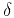) andare allowed to vary. Figure 1 illustrates the concept of the global expansion as we predict the traveltime for anyfrom its behavior atfor the full traveltime field using, in this case, a quadratic approximation. Specifically, we substitute the following trial solution,(4)

where,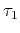, and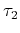are coefficients of the expansion with dimensions of traveltime, into the eikonal equation 3. For practical purposes, I consider here only three terms of the expansion. As a result (as shown in Appendix A),satisfies the eikonal equation for VTI anisotropy, whileandsatisfy linear first-order partial differential equations having the following general form (see Appendix A):(5)

with. The functionsget more complicated for largerand depend on terms that can be evaluated only sequentially. Therefore, these linear partial differential equations must be solved in the order of increasingstarting with.Traveltime approximations for transversely isotropic media with an inhomogeneous backgroundNext: Expansion in terms of Up: Alkhalifah: TI traveltimes in Previous: Introduction

2013-04-02